# Selenium tetrafluoride (SeF4) lewis dot structure, molecular geometry, polar or non-polar, hybridization

Home  > Chemistry Article > SeF4 lewis structure and its molecular geometry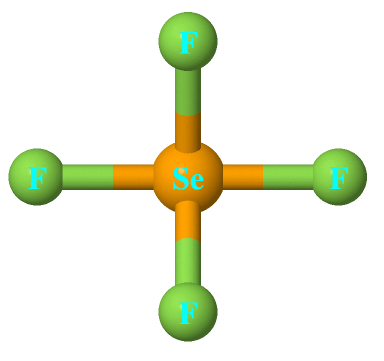Selenium tetrafluoride is an inorganic compound that appears as a colorless liquid having the chemical formula SeF4. It can react with water and forms hydrofluoric acid and selenous acid. Selenium in the SeF4 molecule has a +4 oxidation state.

In this tutorial, we will discuss SeF4 lewis structure, molecular geometry, polar or nonpolar, hybridization, bond angle, etc.

Selenium tetrafluoride is used to add fluorine to other chemical compounds or it acts as a fluorinating agent.

Properties of Selenium tetrafluoride

• It has a molar mass of 154.954 g/mol.
• It appears as a colorless liquid.
• It has a boiling point of 101 °C and a melting point of -13.2 °C.
• Its shape in the gaseous phase is similar to that of SF4
 Name of Molecule Selenium tetrafluoride Chemical formula SeF4 Molecular geometry of SeF4 See-saw Electron geometry of SeF4 Trigonal bipyramidal Hybridization Sp³d Nature polar molecule Total Valence electron for SeF4 34
Page Contents

## How to draw lewis structure for SeF4?

SeF4 lewis structure is made up of one selenium and four fluorine atoms, selenium is the central atom, and fluorine is kept outside in the lewis diagram.

There is one lone pair present on the central atom in the SeF4 lewis structure and 12 lone pairs on outer atoms.

## Follow some steps for drawing the lewis dot structure of SeF4

1. Count total valence electron in SeF4

First of all, determine the valence electron that is available for drawing the lewis structure of SeF4 because the lewis diagram is all about the representation of valence electrons on atoms.

So, an easy way to find the valence electron of atoms in the SeF4 molecule is, just to look at the periodic group of selenium and fluorine atoms.

As selenium atom belongs to the group 16th in periodic table and fluorine situated in 17th group, hence, the valence electron for selenium is 6 and for fluorine atom, it is 7.

⇒ Total number of the valence electrons in selenium = 6

⇒ Total number of the valence electrons in fluorine = 7

∴ Total number of valence electron available for the SeF4 lewis structure = 6 + 7(4) = 34 valence electrons         [∴SeF4 molecule has one selenium and four fluorine atom]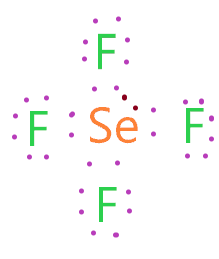2. Find the least electronegative atom and placed it at center

An atom with a less electronegative value is more preferable for the central position in the lewis diagram because they are more prone to share the electrons with surrounding atoms.

In the case of the SeF4 molecule, the selenium atom is less electronegative than the fluorine atom, also, fluorine is the highest electronegative element in chemistry, hence, it always takes the surrounding position in the lewis diagram.

So, just put the selenium in the center and spread all the fluorine atoms around it.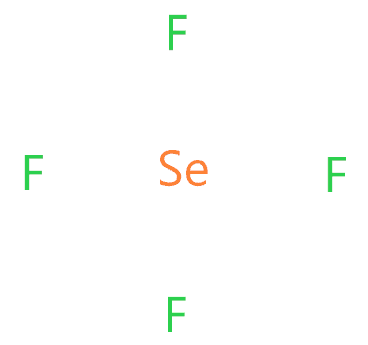3. Connect outer atoms to central atom with a single bond

In this step, place the single bond in between the outer atom(fluorine) and central atom(selenium).After connecting each outer atom to the central atom, count the number of valence electrons used in the above structure. There are 4 single used in the above structure, and one single bond means 2 electrons.

Hence, in the above structure, (4 × 2) = 8 valence electrons are used from a total of 34 valence electrons available.

∴ (34 – 8) = 26 valence electrons

So, we are left with 26 valence electrons more.

4. Place remaining valence electrons starting from outer atom first

Let’s start putting the remaining valence electrons on outer atoms first (in the case of SeF4, fluorine is the outer atom) to complete the octet i.e. Each atom must contain 8 electrons to complete the octet, an exception may occur.So, each fluorine atom in the above structure completed its octet, because all of them have 8 electrons(6 represented as dots and 2 electrons in a single bond) in their outermost shell.

Now again count the number of valence electrons used in the above structure.

There are 6 electrons placed over each fluorine atom, hence, (4 × 6) = 24 valence electrons, and we had 26 valences electrons remaining(check-in 3rd step).

∴ (26 – 24) = 2 valence electrons

So, we are left with only 2 valence electrons.

5. Complete central atom octet and make covalent bond if necessary

In this step, we have to complete the octet of the central atom(selenium), as we already complete the octet of the outer atom(fluorine) in the previous step.

If you look at the 4th step structure, the selenium central atom is attached with 4 single bonds, which means, it has already 8 valence electrons to share. But we have 2 remaining valence electrons that are needed to put somewhere in the lewis diagram.

For this type of problem, a concept comes which is called expanded octet that means an atom can have more than 8 valence electrons.

Selenium is from period 4. Thus, it has d sublevel available. For this reason, selenium can form molecules with expanded octet atoms.

Also, selenium is in the same group on the periodic table as sulfur and thus follows the same pattern of expanded octets.

Hence, selenium central can adopt an extra valence electron due to having an expanded octet ability. So, just put the 2 remaining valence electrons on the selenium atom.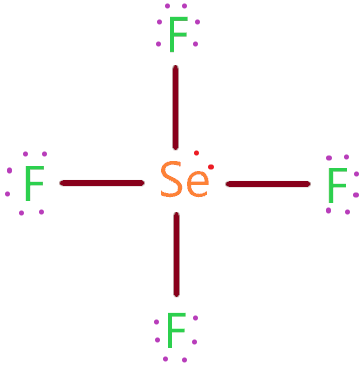### SeF4 lewis structure

So, we used all the valence electrons that are available for drawing the SeF4 lewis structure and both atoms(selenium and fluorine atoms) have completed their octet.

Now just check the stability of the above structure with a formal charge concept.

6. Check the stability with the help of a formal charge concept

The lesser the formal charge on atoms, the better is the stability of the lewis diagram.

To calculate the formal charge on an atom. Use the formula given below-

⇒ Formal charge = (valence electrons – lone pair electrons –  1/2 bonded pair electrons)

Let’s count the formal charge on the fluorine atom, all fluorine atoms in the SeF4 molecule have the same bonded pair and lone pair, so, just count the F.C. for the one fluorine atom.

For fluorine atom:

⇒ Valence electrons of fluorine = 7

⇒ Lone pair electrons on fluorine = 6

⇒ Shared pair electrons around fluorine(1 single bond) = 2

∴ (7 – 6 – 2/2) = 0 formal charge on all fluorine atoms.

For selenium atom

⇒ Valence electrons of selenium = 6

⇒ Lone pair electrons on selenium = 2

⇒ Shared pair electrons around selenium (4 single bonds) = 8

∴ (6 – 2 – 8/2) = o formal charge on the selenium central atom.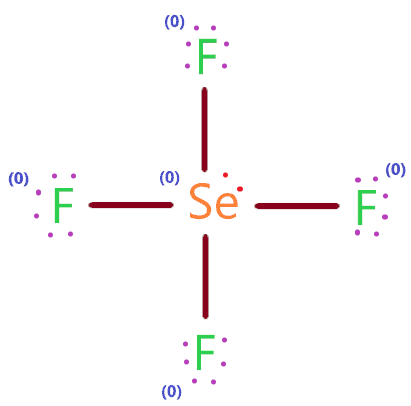So, all the atoms in the SeF4 lewis structure have a formal charge equal to zero. Hence, the above structure is most stable and better.

## What is the molecular geometry of SeF4?

The molecular geometry of SeF4 is see-saw and electron geometry is trigonal bipyramidal, this is because the selenium central atom has one lone pair on equatorial position and 4 bonded pairs are attached to it.

As per VSEPR theory, the lone pair on the central atom tries to repel the bonded pair of electrons around it, as a result, all electrons are pushed far apart from each other, hence, distorted the final shape of the molecule.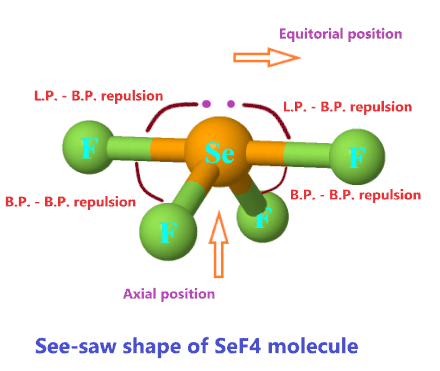As you see in the above picture, the presence of lone pair on a selenium atom repels strongly adjacent bonded pair electrons, and same as, bond pair electrons repels the other adjacent bonded pair electrons. Hence, all electrons pushing each other.

But the repulsion of the lone pair is stronger than bonded pair, as a result, all atoms are set in a see-saw position. “The name “seesaw” comes from the observation that it looks like a playground seesaw.”

So, the final molecular geometry of SeF4 is see-saw in shape.

If you find difficulty in visualizing how electron pairs repulsion works in molecular shape, then you can find the shape of any molecule with the AXN method.

AXN is the generic formula of electron pairs(Bond pairs + Lone pairs) around a central atom. It predicts the shape or geometry of the molecule by taking the help of the VSEPR chart.

Let’s find the molecular shape and electron geometry of SeF4 using the AXN method.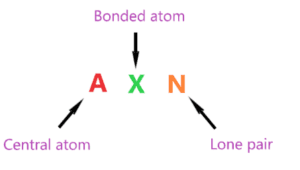AXN notation for SeF4 molecule:

• A denotes the central atom, so, in the SeF4 molecule, selenium is the central atom. A = Selenium
• X denotes the bonded atoms to the central atom, as we know, selenium is bonded with four fluorine atoms. Therefore, X = 4
• N denotes the lone pair on the central atom, as per SeF4 lewis structure, selenium has only one lone pair. Hence, N = 1

So, the AXN generic formula for the SeF4 molecule becomes AX4N1.

As per the VSEPR chart, if a molecule central atom is attached with four bonded atoms and has one lone pair then the molecular geometry of that molecule is see-saw in nature, and electron geometry is trigonal bipyramidal.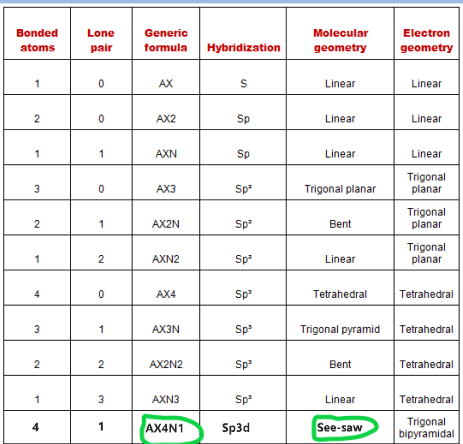Hence, the molecular shape for SeF4 is see-saw and its electron geometry is trigonal bipyramidal.## Hybridization of SeF4

Let’s find the hybridization of SeF4 through the steric number of its central atom.

“Steric number is the addition of a total number of bonded atoms around a central atom and the lone pair present on it.”

∴ Steric number of SeF4 = (Number of bonded atoms attached to selenium + Lone pair on selenium)

According to the SeF4 lewis structure, selenium is bonded with four fluorine atoms and it contains one lone pair also.

∴ Steric number of SeF4 = (4 + 1) = 5

 Steric number Hybridization 1 S 2 Sp 3 Sp² 4 Sp³ 5 Sp³d 6 Sp³d²

So, for a steric number of five, we get the Sp3d hybridization on selenium atom in SeF4 molecule.

“One s orbital, three p orbitals and one d orbital hybridize to produce five sp3d hybrid orbits. In four of these orbitals, bond pair electrons are present. In the fifth orbital, lone pair of electrons resides.”

## The bond angle of SeF4

“The axial Se-F bonds are 177 pm with an F-Se-F bond angle of 169.2°. The two other fluorine atoms are attached by shorter bonds (168 pm), with an F-Se-F bond angle of 100.6°.”

## Selenium tetrafluoride polarity: is SeF4 polar or nonpolar?

So, Is SeF4 polar or nonpolar? SeF4 is a polar molecule because of its asymmetrical structure which causes uneven distribution of charges in the molecule, thus, dipole moments generated along with the bonds are unable to cancel out each other, making SeF4 a polar molecule in nature.

Also, in the SeF4 molecule, fluorine is more electronegative than selenium, so, the electronegativity difference between these atoms makes the bond polar(F-Se).

However, the molecular shape of SeF4 is a see-saw, so, two fluorine atoms in opposite directions situated on equatorial position cancel out each other charges because of the same electronegativity differences of (F-Se) bond on both sides.

But the two fluorine that is situated below to lone pair and makes the 120º angle, maintained their charges, as both of them are in the same direction, hence, charges along these cannot cancel out.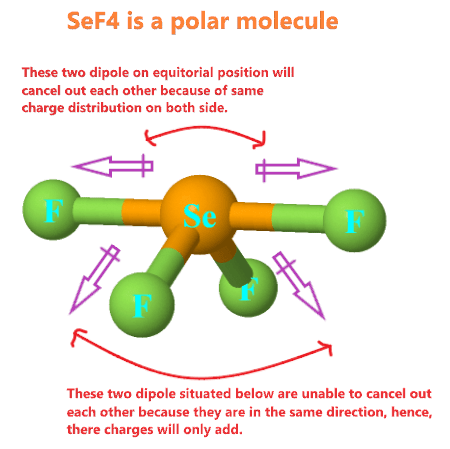So, these two Se-F bonds that are pointing down, make the SeF4 molecule polar in nature.

## FAQ

### Why is the molecule geometry of SeF4 is see-saw but not a trigonal bipyramidal?

Both see-saw and trigonal bipyramidal have a number of regions of electron density(5), both form the same hybridization.

But trigonal bipyramidal geometry has no lone pair on the central atom, means, it only contains 5 bonded atom that makes generic formula AX5.

Whereas see-saw geometry has 1 lone pair on the central atom and 4 bonded atom that makes the generic formula AX4N1.

So, as per the SeF4 lewis structure, the selenium central atom has one lone pair and is attached with 4 bonded atoms, hence, it forms a see-saw shape according to the VSEPR theory.

### How many lone pair and bond pair electrons are present around the central atom in the SeF4 lewis structure?

By looking at the SeF4 lewis structure, we see that selenium is the central atom that contains 2 lone pair electrons and it has 8 bond pair electrons(4 single bonds) around.

## Summary

• The total valence electron is available for drawing the SeF4 lewis structure is 34.
• The steric number of selenium central atom in the SeF4 molecule is 5, thus, it forms Sp3d hybridization.
• SeF4 is a polar molecule because of asymmetrical geometry that causes the non-uniform distribution of charge in the molecule.
• In the SeF4 lewis structure, a total of 13 lone pairs and 4 bond pairs are present.
• The molecular geometry of SeF4 is see-saw and electron geometry is trigonal bipyramidal.
##### Subscribe to Blog via Email

Join 2 other subscribers

Share it...